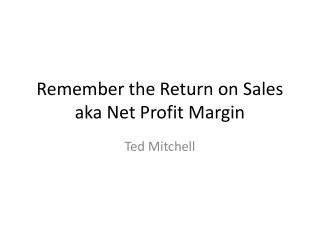DownloadDownload PresentationRemember the Return on Sales aka Net Profit Margin

# Remember the Return on Sales aka Net Profit Margin

Télécharger la présentation## Remember the Return on Sales aka Net Profit Margin

- - - - - - - - - - - - - - - - - - - - - - - - - - - E N D - - - - - - - - - - - - - - - - - - - - - - - - - - -
##### Presentation Transcript

1. Definitions of ROS are • If I ask you for a definition of the • Return on Sales (ROS) • What do you remember as the equation?

2. Definitions of ROS are • ROS = Z/R where Z = G-E • ROS = (G-E)/R where G = R-COGS • ROS = ((R-COGS) – E)/R where R = P(Q), COGS = V(Q) • ROS = (P(Q) – V(Q) – E)/R where G = Mp(R) • ROS = (Mp(R) – E) /R where Mp = (P-V)/P • Where Z= profit after promotion expense, E = promotion expense, G = gross profit.R= sales revenue, COGS = cost of goods sold, P=selling price, Q= quantity sold, V=variable cost per unit, Mp = markup on price

3. How do we use ROS? • 1) For Predicting the profit, Z, with a forecasted revenue, R*, knowing the normal and expected ROS Z = ROS x R* 2) Calculating the selling price, P, knowing the normal and expected breakeven price, BEP, the normal and fair, ROS, the normal hurdle rate or IRR (i.e., ROME) required from marketing P = BEP/(1-ROS)

4. How do we interpret ROS? • ROS is a measure of the average rate of efficiency or effectiveness of the marketing department converting Revenue into the Profit after Promotion • In Setting the selling price, ROS is the Profit Returned by Sales and it is a popular way to rank the market power of the product’s sales in the market

5. The Roots of ROS are • 1) It is a cost-based method for Setting the selling based on the basic profit equation Z = P(Q) – V(Q) – E P = V + (E/Q) + Z/Q • 2) Breakeven Price, BEP, is where Z = 0BEP = V + E/Q where V + (E/Q) is the average cost per unit • 3) Setting the selling price, P, by defining the profit it must bring inZ = ROS x R P = (V + E/Q) + ROS(R)/Q where R = P(Q) P = BEQ/(1-ROS)

6. The Virtues are • 1) Simple to calculate • 2) Fair: passes cost increases to the customer • 3) A basis for Industry stability given similar costs • 4) Guarantees a profit in normal, fair and expected times

7. The Weaknesses of ROS are • 1) assumes that forecasted revenue is independent of the chosen selling price. • 2) it is not fair in that it leads to passing a additional percentage of all costs to the customer for covering the normal, fair and expected profit. • 3) it leads to implicit price collusion

8. Remember the Return on Sales, ROS